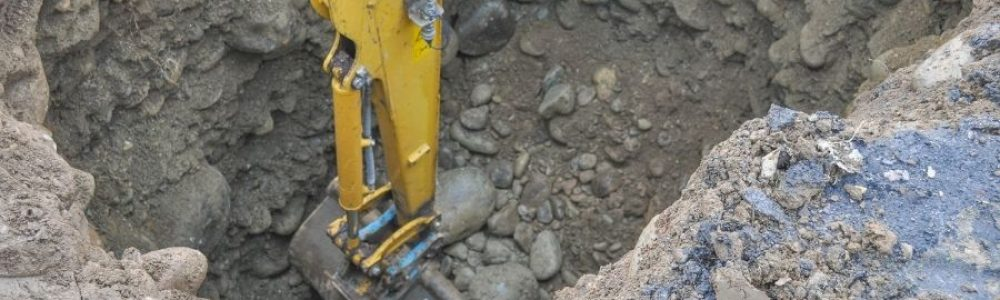# How to Figure Out How Many Yards of Dirt I Need

## Cubic Yard to “Yard” Calculations

When landscaping your home, you will need to determine how much dirt you need to complete the project. To figure this out, you will need to measure the length and width of the area you are working on. Once you have these measurements, you can use a simple equation to calculate the total square feet. Next, divide the square feet by 43, which is the number of cubic feet in a Yard. This will give you the number of yards you will need for your project.

For a clear explanation of how to do these calculations, watch this great. short video by Forest To Farm.